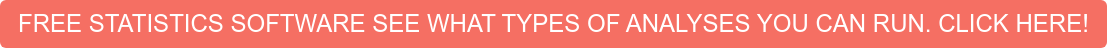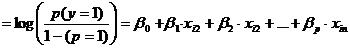# What is Logistic Regression?

Logistic regression is the appropriate regression analysis to conduct when the dependent variable is dichotomous (binary).  Like all regression analyses, the logistic regression is a predictive analysis.  Logistic regression is used to describe data and to explain the relationship between one dependent binary variable and one or more nominal, ordinal, interval or ratio-level independent variables.

Sometimes logistic regressions are difficult to interpret; the Intellectus Statistics tool easily allows you to conduct the analysis, then in plain English interprets the output.

# Quantitative Results in One-HourScreen share with a statistician as we walk you through conducting and understanding your interpreted analysis. Have your results draft complete in one hour with guaranteed accuracy.Type of questions that a binary logistic regression can examine.

How does the probability of getting lung cancer (yes vs. no) change for every additional pound a person is overweight and for every pack of cigarettes smoked per day?

Do body weight, calorie intake, fat intake, and age have an influence on the probability of having a heart attack (yes vs. no)?

Binary logistic regression major assumptions:

1. The dependent variable should be dichotomous in nature (e.g., presence vs. absent).
2. There should be no outliers in the data, which can be assessed by converting the continuous predictors to standardized scores, and removing values below -3.29 or greater than 3.29.
3. There should be no high correlations (multicollinearity) among the predictors.  This can be assessed by a correlation matrix among the predictors. Tabachnick and Fidell (2013) suggest that as long correlation coefficients among independent variables are less than 0.90 the assumption is met.

At the center of the logistic regression analysis is the task estimating the log odds of an event.  Mathematically, logistic regression estimates a multiple linear regression function defined as:

logit(p)for i = 1…n .

Overfitting.  When selecting the model for the logistic regression analysis, another important consideration is the model fit.  Adding independent variables to a logistic regression model will always increase the amount of variance explained in the log odds (typically expressed as R²).  However, adding more and more variables to the model can result in overfitting, which reduces the generalizability of the model beyond the data on which the model is fit.

Reporting the R2.  Numerous pseudo-R2 values have been developed for binary logistic regression.  These should be interpreted with extreme caution as they have many computational issues which cause them to be artificially high or low.  A better approach is to present any of the goodness of fit tests available; Hosmer-Lemeshow is a commonly used measure of goodness of fit based on the Chi-square test.

Statistics Solutions or the Intellectus Statistics software can assist with your quantitative analysis by editing your methodology and results chapters.*Please call 877-437-8622 to request a quote based on the specifics of your research, or email Info@StatisticsSolutions.com.

Related Pages:

Conduct and Interpret a Logistic Regression

Assumptions of Logistic Regression